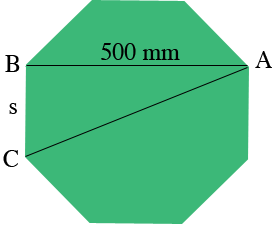SEARCH HOMEMath Central Quandaries & QueriesQuestion from Joe: If the dimension from one flat part of an octagon to opposite flat is 500 mm what is the largest diameter (point to point) of the octagon?Hi Joe,

I drew a diagram of your regular octagon.If $d$ is the distance from $A$ to $B,$ in your example 500 mm, and $s$ is the side length, the distance from $B$ to $C$ then in a response to a question from Lewis Penny showed that

$d = \left( 1 + \sqrt{2} \right) s.$

Use her expression to find $s$ for your octagon.

Triangle $ABC$ is a right triangle so you can use Pythagoras Theorem to find the distance from $C$ to $A.$

Write back if you need more assistance,

HarleyMath Central is supported by the University of Regina and The Pacific Institute for the Mathematical Sciences.